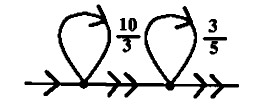## Fractran is based on one of the most bizarrely elegant concepts of computation.

A Fractran program is an ordered list of positive fractions together with an initial positive integer input. The program is run by updating the accumulator.

Any number that can't be divided by any other number, apart from itself and one, is prime. Since primes can't be divided, we can think of them as the DNA of other numbers. In Fractran, each prime is a register and their exponent is their value.

### The Accumulator

The state of the accumulator is held as a single number, whose prime factorization holds these registers(2, 3, 5, 7, 11, 13, 17, ..). If the state of the accumulator is 1008(2⁴ × 3² × 7), r2 has the value 4, r3 has the value 2, r7 has the value 1, and all other registers are unassigned.

AccumulatorRegisters
r2r3r5r7
611
1812
1008421
54022501234

### The OperatorsA fractran operation is a positive fraction, each fraction represents an instruction that tests one or more registers, represented by the prime factors of its denominator. The Fractran computer goes through each fraction in order, in terms of our current accumulator value.

`18(21 × 32) 2/3 = 8(23) addition r2+r3->r2`

To run the adder operation(`2/3`), we will take the state of the accumulator. If multiplying it by this fraction will give us an integer, we will do so and start again at the beginning of the program. Otherwise, we will stop and consider the program complete. We will do this repeatedly until we can no longer produce an integer with this method.

stepsstateregisters
r2r3
1181218 × 2/3 = 12/1INT, RESTART
2122112 × 2/3 = 8/1INT, RESTART
3838 × 2/3 = 16/3NOT INT, END

To add the values 1 and 2, we will store the values in registers 2 and 3, our starting state is therefore 18(21 × 32).

For each step of the program, we will multiply our state with the program(18 × 2/3 = 12, 12 × 2/3 = 8, ..) until our our working value cannot be reduced to a whole number(16/3), we have exhausted the program. Alternatively, the program `3/2` will do the same operation but store the result in the register 3.

`576(26 × 32) 1/6 = 16(24) subtraction r2-r3->r2`

Operations become more readable when broken down into their primes. We can think of every prime number as having a register which can take on non-negative integer values. Each fraction is an instruction that operates on some of the registers.

2/3 15/256 21/20
(21)/(31) (31 × 51)/(26) (31 × 71)/(22 × 51)
```if(r3 >= 1){
r3 -= 1;
r2 += 1;
return;
}```
```if(r2 >= 6){
r2 -= 6;
r3 += 1;
r5 += 1;
return;
}```
```if(r2 >= 2 && r5 >= 1){
r2 -= 2;
r5 -= 1;
r3 += 1;
r7 += 1;
return;
}```

You can interpret a fraction as saying if the current value of each register is greater than or equal to the value specified by the denominator, you subtract from the registers all of the values in the denominator, add all the values specified in the numerator, and then jump back to the first instruction. Otherwise, if any register is less than the value specified in the denominator, continue to the next fraction.

### The ProgramsA Fractran program is a list of fractions together with an initial positive integer input n. The program is run by updating the integer n as follows:

• For each fraction in the list for which the multiplication of the accumulator and the fraction is an integer, replace the accumulator by the result of that multiplication.
• Repeat this rule until no fraction in the list produces an integer when multiplied by the accumulator, then halt.

Let's put together an adder program similar from the one above(`2/3`) but which writes to a third register. The following program first moves the content in r2 to r3, and then the content of r3 to r5.

`18(21 × 32) 3/2 5/3 = 125(53) addition r2+r3->r5(9 steps)`

Alternatively, a faster way to do this would be to directly move powers of 2 over to 5, then powers of 3.

`18(21 × 32) 5/2 5/3 = 125(53) addition r2+r3->r5(7 steps)`

Each of the 7 steps of this last program looks like:

```18 5/2 5/3             r2=01 r3=02
------------------   -----------------
18 × 5/2 = 45/1        r3=02 r5=01
45 × 5/2 = 225/2
45 × 5/3 = 75/1        r3=01 r5=02
75 × 5/2 = 375/2
75 × 5/3 = 125/1      r5=03
125 × 5/2 = 625/2
125 × 5/3 = 625/3     r5=03
```

Both of these programs are destructive, meaning that they drain the registers of their original values. We can make (`2/3`) less destructive with (`10/3`) by storing a copy of r3 in r5. And we can create a non-destructive adder but this requires coming in with the program with the flag r7 set:

`126(21 × 32 × 71) 7/11 715/14 935/21 1/7 2/13 3/17 = 2250(21 × 32 × 53)`

As an extra demonstration, let us consider the following programs representing all the logic gates:

 Program AND Gate OR Gate XOR Gate `07` `14` `21` `42` `5/42 1/21 1/14 1/7` 1 1 1 5 `5/42 5/21 5/14 1/7` 1 5 5 5 `1/42 5/21 5/14 1/7` 1 5 5 1 `1/42 5/21 5/14 5/7` 5 5 5 1 `1/42 1/21 1/14 5/7` 5 1 1 1 `5/42 1/21 1/14 5/7` 5 1 1 5

### Extras

I've been scratching at trying to fit a fractran-inspired vm hosted in uxn's 16-bit space where these fractions operate differently to facilitate running with low values in registers.

`*/*` Jump Push Acc/Load from memory Pop Acc/Save in memory Pop, or Break
A wise person marvels at the commonplace. Confucius

incoming phutball firth primes fractions